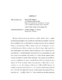## SOLAR NEUTRINOS AT SUPER-KAMIOKANDE: SOLVING THE SOLAR NEUTRINO PUZZLE VIA NEUTRINO FLAVOR OSCILLATIONS##### Files
dissertation.pdf(2.48 MB)
The Super-Kamiokande neutrino detector was built with the intent to explain the long-standing apparent solar neutrino flux deficit through signatures of neutrino flavor oscillations, such as a distortion in the energy spectrum and an asymmetry in the day and night fluxes. With the absence of any such smoking-gun'' evidence, an oscillation analysis of solar neutrinos was performed using the data sample from Super-Kamiokande I (SK), Sudbury Neutrino Observatory (SNO), and all other neutrino detectors. A model-independent analysis of SK's total solar neutrino rate and SNO's solar electron-neutrino rate showed at $3.7\,\sigma$ level that the apparent deficit is due to the effects of neutrino flavor oscillations. This analysis was possible because for a careful choice of energy thresholds, SK and SNO have virtually the same response to $^8$B solar neutrinos, whose energy spectrum is undistorted, as demonstrated by the data. By utilizing the full data sets of SK and SNO, however, the oscillation scenario is favored at $6.0\,\sigma$ level, with the best-fit oscillation parameters of $\Delta m^2=6.3\times10^{-5}\rm\,eV^2$ and $tan^2\theta=0.44$ (in the LMA region). The measured $^8$B neutrino flux is $\Phi_\nu=5.45^{+0.64}_{-0.69} \times10^6\rm\,cm^{-2}s^{-1}$, which confirms its theoretical prediction from the Standard Solar Model. With the addition of the neutrino rates from the radiochemical experiments (gallium and chlorine), and the anti-neutrino oscillation result from KamLAND, the LMA solution is further constricted, the $^8$B neutrino flux is again confirmed ($\Phi_\nu=5.66^{+0.62}_{-0.59} \times10^6\rm\,cm^{-2}s^{-1}$), and the no-oscillation scenario is ruled out at more than $10\,\sigma$ level.